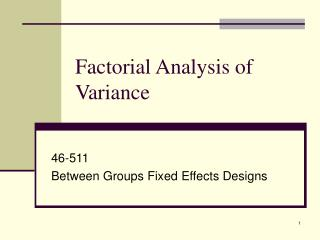Download PresentationFactorial Analysis of Variance

# Factorial Analysis of Variance - PowerPoint PPT Presentation

Factorial Analysis of Variance. 46-511 Between Groups Fixed Effects Designs. Two-Way ANOVA Example: (Yerkes – Dodson Law). Factor B: Arousal. Factor A: Task Difficulty. Partitioning Variance. Factor B: Arousal. Variation among means on A represent effect of A. Factor A: Task Difficulty.I am the owner, or an agent authorized to act on behalf of the owner, of the copyrighted work described.
Download Presentation## Factorial Analysis of Variance

An Image/Link below is provided (as is) to download presentation

Download Policy: Content on the Website is provided to you AS IS for your information and personal use and may not be sold / licensed / shared on other websites without getting consent from its author.While downloading, if for some reason you are not able to download a presentation, the publisher may have deleted the file from their server.

- - - - - - - - - - - - - - - - - - - - - - - - - - E N D - - - - - - - - - - - - - - - - - - - - - - - - - -
Presentation Transcript
1. Factorial Analysis of Variance 46-511 Between Groups Fixed Effects Designs

2. Two-Way ANOVA Example:(Yerkes – Dodson Law) Factor B: Arousal Factor A: Task Difficulty

3. Partitioning Variance Factor B: Arousal Variation among means on A represent effect of A Factor A: Task Difficulty Variation among people treated the same = error Variation among means on B represents effect of B Leftover variation = interaction

4. Partitioning Variance: Interaction Factor B: Arousal Factor A: Task Difficulty Dependence of means on levels of both A & B represents the effect of an interaction.

5. Or Graphically…

6. In words • Types of Effects vs. 1-way • Main Effect for A • Main Effect for B • Interaction (A x B) • Structural Model: XIJK = μ++++IJK • Partitioning Variance/Sums of Squares • First, total variance: • Between Groups: • Thus Total is:

7. Sums of Squares Between Definitional Formula Variation of cell means around grand mean, weighted by n. Computational Formula • Computational formulae: • More accurate for hand calculation • Easier to work • Less intuitive

8. Sums of Squares A Definitional Formula Variation of row means around grand mean, weighted by n times the number of levels of B, or q. Computational Formula

9. Sums of Squares B Definitional Formula Variation of column means around grand mean, weighted by n times the number of levels of A, or p. Computational Formula

10. Sums of Squares AxB Definitional Formula Computational Formula SSAxB = Variation of cell means around grand mean, that cannot be accounted for by effects of A or B alone.

11. Sums of Squares Within (Error) Definitional Formula Computational Formula SSW = Variation of individual scores around cell mean.

12. Numerical Example

13. Degrees of Freedom • df between = k – 1; or, (kA x kB – 1) • df A = kA – 1 • df B = kB – 1 • df A x B = dfbetween – dfA – dfB • dfW = k(n-1)

14. Source Table

15. More Digression on Interactions • Ways to talk about interactions • Scores on the DV depend upon levels of both A and B • The effect of A is moderated by B • The effect of B is moderated by A • There is a multiplicative effect for A and B

16. More Digresions (cont’d)No effect whatsoever…

17. Main effects for A and B…

18. Graphically…

19. Interaction significant also…

20. Graphically…

21. Further Analyses on Main Effects • Contrasts • Planned Comparisons • Post-Hoc Methods • In the presence of a significant interaction

22. Further Analyses on Interaction • What it means • Simple (Main) Effects • Contrasts • Partial Interactions • Contrasts • Simple Comparisons / Post-Hoc Methods • How to get q

23. Simple Main Effects Analysis

24. Simple Main Effects Sum of Squares Formula: F Ratio: df = dfj,dfw:

25. Partial Interaction Analysis

26. In Class Exercise

27. Effects A B C A x B A x C B x C A x B x C A Vague Example DV = Treatment Outcome Factor A: Gender Factor B: Age (14 or 17) Factor C: Treatment 3-Way ANOVA

28. Results

29. Significant Two-Way Interaction

30. Significant Three-Way Interaction

31. Other Stuff • Higher order models (4-way, 5-way, etc.) • Unequal Cell Sizes and SS Type • Use of contrast coefficients • Short-Cuts using SPSS • Custom Models in SPSS • Observed Power